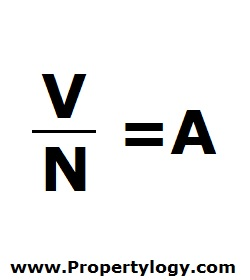Formula - Moving Average | Propertylogy
Don't Miss

# Formula – Moving Average

By on June 12, 2018V/N = A

V – Sum of values

N – Number of values

A – Moving average

To find the moving average of real estate values, there is first a need to determine the field of value and the period in question.

For example if the field of value is month-end average property value and the period is 2 years, there would be a total of 24 average property values too add up.

V would therefore be the sum of all 24 values, and N would be 24.

In investment analysis, moving average allows an analyst to see how well the market is doing currently in comparison to the average over the specified period of time.

You May Also Like...##### How to burn more calories in the office

Send this to a friend